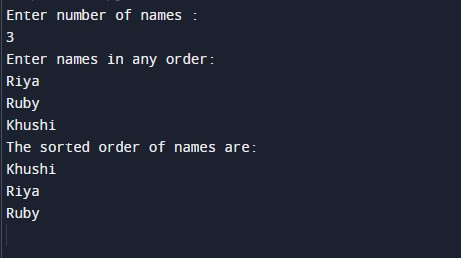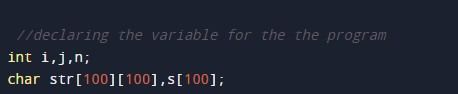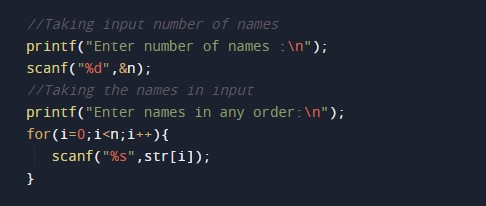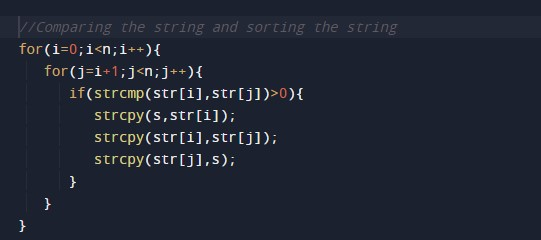C Program to Sort Names in Alphabetical Order

In this tutorial you will learn about the C Program to Sort Names in Alphabetical Order and its application with practical example.

C Program to Sort Names in Alphabetical Order

In this tutorial, we will learn to create a C program that will Sort Names in Alphabetical Order using C programming.

Prerequisites

Before starting with this tutorial we assume that you are best aware of the following C programming topics:

• Operators in C Programming.
• Basic Input and Output function in C Programming.
• Basic C programming.
• For loop in C Programming.
• String functions in C programming.
• Conditional statement in C Programming.

Sort Names in Alphabetical Order:-

As we all know String is a collection of similar data type elements. In an array, only one variable is declared which can store multiple values. First will take the string input from the user. Then will pass that string to the for loop to sort it in alphabetical order. And at last, we will Sort Names in Alphabetical Order C Programming Language.

Program:-

Sort Names in Alphabetical Order

Output:-In the above program, we have first initialized the required variable• s = it will hold the elements in a string.
• str = it will hold the string value.
• i = it will hold the integer value to control the array.
• j = it will hold the integer value to control the array.
• n = it will hold the integer value to control the array.

Taking input string from the user.Sorting the string in alphabetical order.Printing the results.In this tutorial we have learn about the C Program to Sort Names in Alphabetical Order and its application with practical example. I hope you will like this tutorial.